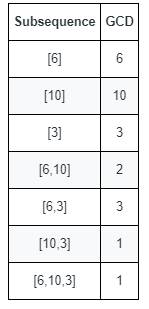# LeetCode 1819. 序列中不同最大公约数的数目

## 1. 题目输入：nums = [6,10,3]

1 <= nums.length <= 10^5
1 <= nums[i] <= 2 * 10^5


## 2. 解题

• 不能枚举子序列，2^n 很大
• 枚举 最大公约数 g ，参考题解区
class Solution {
public:
int countDifferentSubsequenceGCDs(vector<int>& nums) {
int maxnum = *max_element(nums.begin(), nums.end());
vector<int> hasnum(maxnum+1, false);//数字是否存在
for(auto n : nums)
{
hasnum[n] = true;
}
int count = 0;
for(int g = 1; g <= maxnum; g++)//枚举最大公约数
{
int gcd = -1;//实际的公约数
for(int num = g; num <= maxnum; num += g)
{   // 枚举 公约数 g 的倍数的 num
if(hasnum[num])// num 存在
{
if(gcd == -1)
gcd = num;
else
gcd = __gcd(num, gcd);//序列的最大公约数
if(gcd <= g)// = 找到了序列的 最大公约数 gcd 为 g
// < gcd 不会变大, 停止搜索
break;
}
}
if(gcd == g)
count++;
}
return count;
}
};


328 ms 95.9 MB C++07-13146704-0427
03-133517
10-08786
04-103
04-1015
04-0418
04-0816
04-11164
04-0436
01-1414
12-2061
03-274万+
02-0683
02-278
01-10364
01-1412
05-27105
04-0959
©️2020 CSDN 皮肤主题: Age of Ai 设计师:meimeiellie